## Monday, 16 February 2015

### My Favourite Mathematicians

Continuing the 'My favourite...' series, I will now describe a few of my favourite past and present mathematicians. Due to the extreme number of extremely good mathematicians, this list was especially hard to form but I think I have narrowed down my favourite five.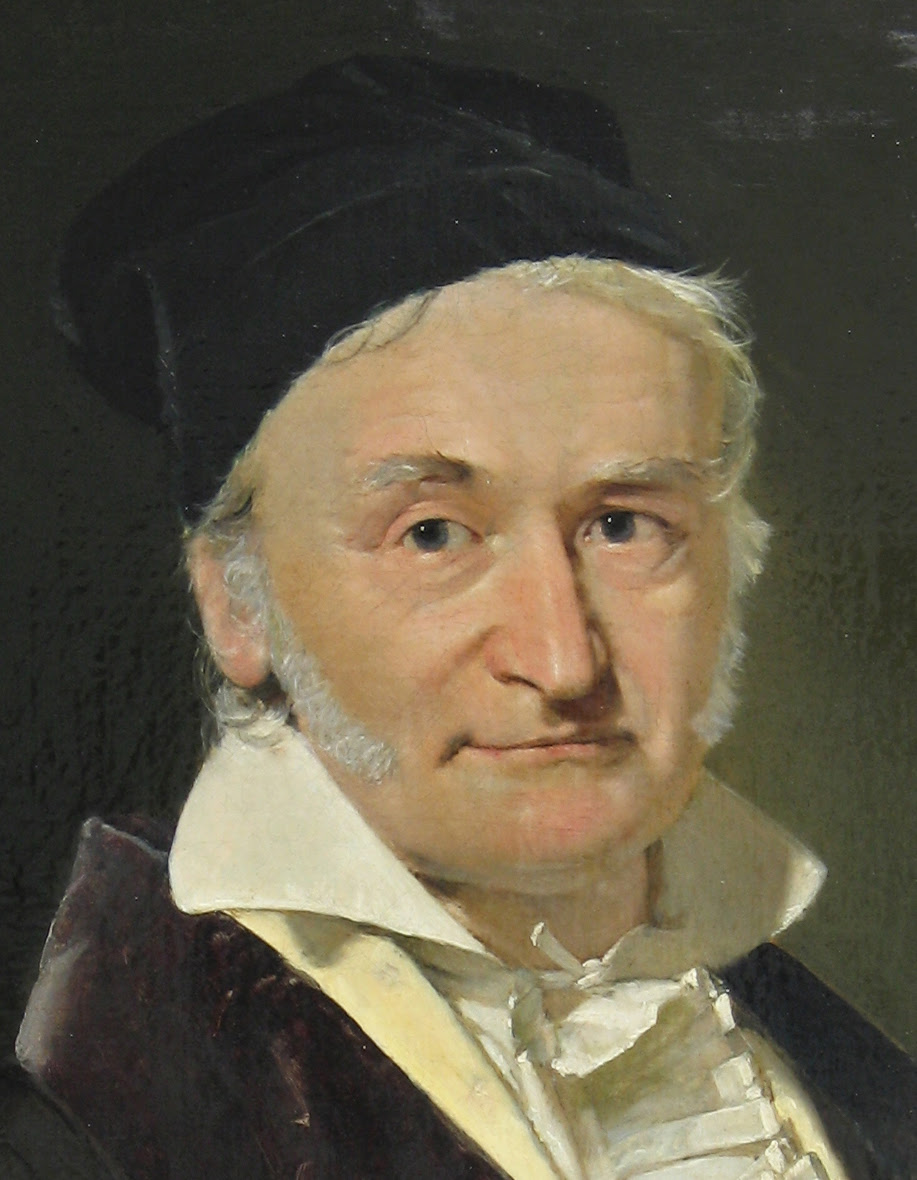Carl Friedrich Gauss
A truly incomparable mathematician who had a major influence in various areas of maths even at a very young age. I cannot name even a fraction of his various contributions to mathematics but they include:

Suffice it to say, he has had an incredible influence in almost all areas of maths since the 18th century.

Euclid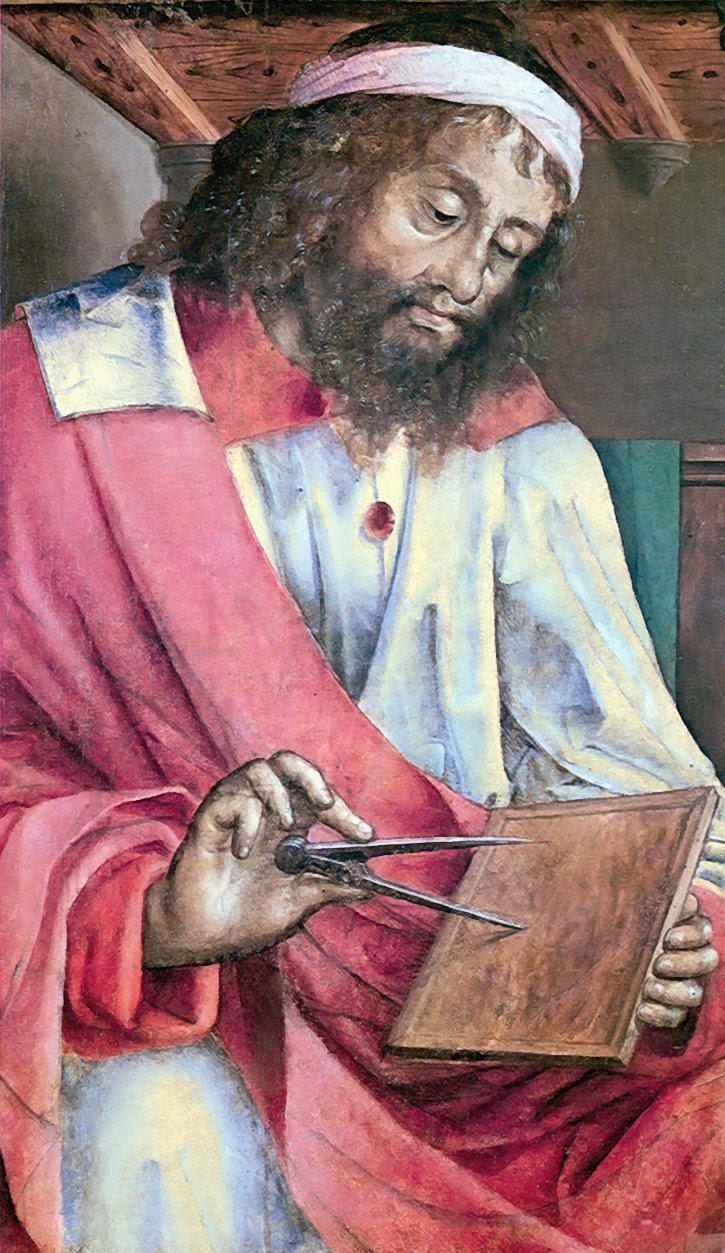Euclid, the classic Greek mathematician, was a person who revolutionised maths, introducing and developing whole fields of mathematics, especially geometry (he is known as the 'Father of Geometry') and axiomatic theory.
His most prominent work, the Elements, set the way for maths in the following millenia and until the 21st century was the second most sold work worldwide, following only the Bible.

One of his most important ideas he put into practice was the use of axioms, specifically the 5 Postulates from which all his proofs came:
1. A straight line segment can be drawn joining any two points.
2. Any straight line segment can be extended indefinitely in a straight line.
3. Given any straight line segment, a circle can be drawn having the segment as radius and one endpoint as center.
4. All right angles are congruent.
5. If two lines are drawn which intersect a third in such a way that the sum of the inner angles on one side is less than two right angles, then the two lines inevitably must intersect each other on that side if extended far enough. This postulate is equivalent to what is known as the parallel postulate.

You'll note that the 5th is rather more complex than the other four, but that is also a story for another post...

Leonhard Euler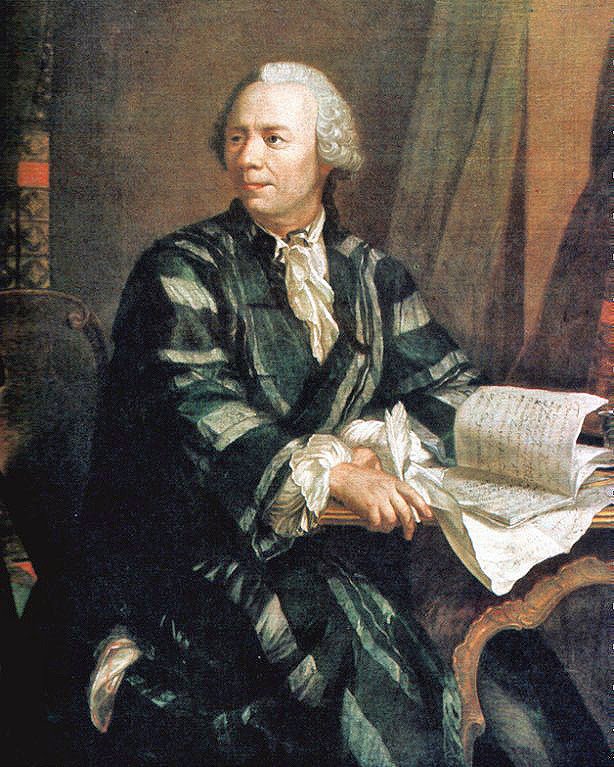I don't know whether I can put this better than Laplace when he said:
"Read Euler, read Euler, he is the master of us all."
Euler made great discoveries, like all in this list, in various areas of maths but there is one which I associate most strongly with Euler: Euler's Identity.
Euler's identity is what some, if not most, mathematicians call the greatest work of maths as it links seemingly distinct areas of maths in a wonderfully simple and concise equation: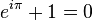$e^{i \pi} + 1 = 0$
where:

• 'e' is the base of the natural logarithm, fundamental in calculus and discovered by Gauss to be linked strongly with the primes
• 'π' is the ratio between the circumference and diameter of a circle, fundamental to Euclidean and non-Euclidean geometry (named after Euclid of course)
• 1 is the multiplicitive identity, i.e. anything multiplied by 1 is itself
• 0 is the additive identity, i.e. anything add 0 is itself

This simple equation links all of these together and is in my opinion the greatest result in mathematics ever.

Pierre de Fermat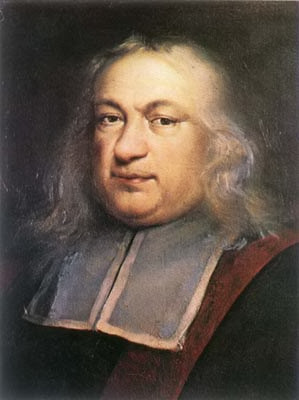Unlike the others in this list, Fermat was an amateur mathematician but, as E.T. Bell put it, the 'Prince of Amateurs'.
He was most famous probably for not what he did, but what he didn't do. He was famed for claiming he had solved big problems without releasing his proofs, most famously in the case of 'Fermat's Last Theorem':
"It is impossible to separate a cube into two cubes, or a fourth power into two fourth powers, or in general, any power higher than the second, into two like powers. I have discovered a truly marvellous proof of this, which this margin is too narrow to contain."

The jury is still out as to whether he actually solved it or not, but given that it was only solved 358 years later using areas of mathematics as yet undiscovered, it seems unlikely.
However that does not detract from his brilliance as a mathematician.

Srinivasa Ramanujan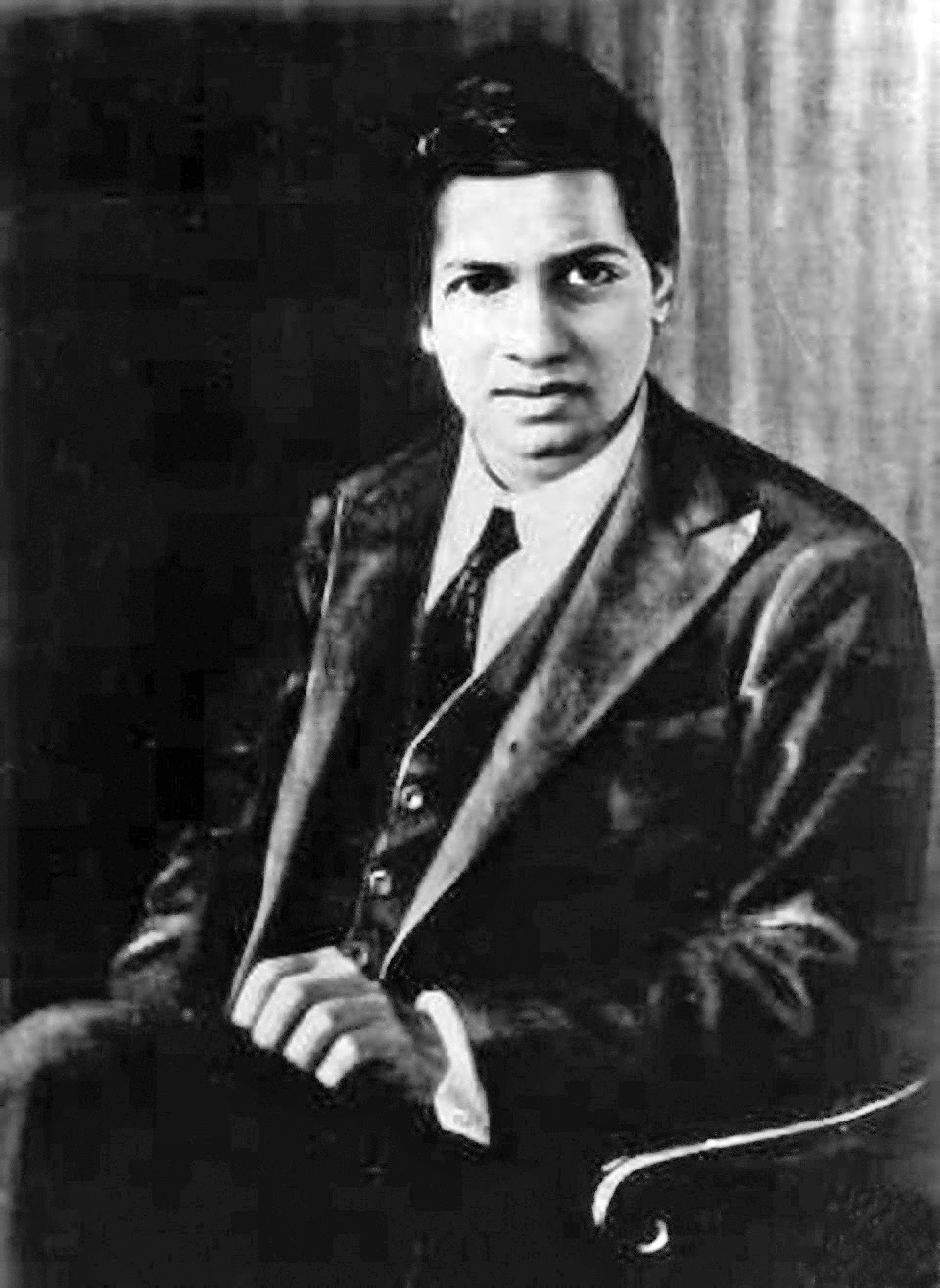Ramanujan, the most modern mathematician in this list, was an exceptional person. Untrained, self-taught and a bit mad (who isn't?), he was brought over to England by G. H. Hardy, author of A Mathematician's Apology, after the exchange of a number of letters which revealed Ramanujan to be a truly incredible mathematician.
In his comparatively short mathematical life, he came up with around 3900 results notably making progress towards areas of maths including infinite series, continued fractions,...
As Erdős recollected, "Suppose that we rate mathematicians on the basis of pure talent on a scale from 0 to 100, Hardy gave himself a score of 25, J.E. Littlewood 30, David Hilbert 80 and Ramanujan 100."

But what he is now most famous for outside of mathematical circles is the taxicab story, which highlights the brilliance of this man:
"I remember once going to see him when he was ill at Putney. I had ridden in taxi cab number 1729 and remarked that the number seemed to me rather a dull one, and that I hoped it was not an unfavorable omen. "No", he replied, "it is a very interesting number; it is the smallest number expressible as the sum of two cubes in two different ways."

A few others:
• Blaise Pascal
• Pythagoras
• Archimedes
• Isaac Newton
• Gottfried Wilhelm Leibniz
• René Descartes
• David Hilbert
• Évariste Galois
• Kurt Gödel
• Andrew Wiles

## Tuesday, 10 February 2015

### My Favourite Maths Books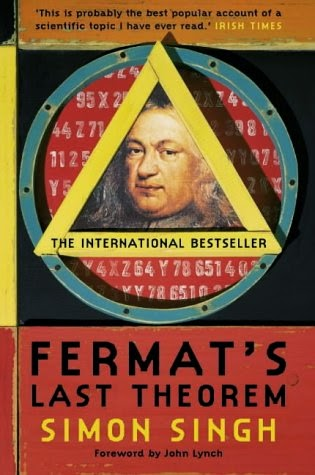I have a (relatively) large selection of maths books which I really enjoy reading and which were what I would say sparked my love for maths. I would love to share with you my top 5 of these.

1) Fermat’s Last Theorem by Simon Singh
This is the book that really kick-started my love for maths. It provides a great history of maths from the Greeks to modern days whilst keeping the running theme of Fermat’s Last Theorem. It was the first book to introduce me to the ideas of proof, I read it when I was 11 or 12, and it really left an imprint in my mind about what maths is all about.It also describes the actual problem extremely well, and shows its difficulty through the vast number of mathematicians attempting it over the 358 years it was unsolved. It is definitely my favourite maths book and will always have a place in my heart.

2) Alex’s Adventures in Numberland by Alex Bellos
Another book which has shaped my mathematical journey is this one. It contains such a great variety of information, from ancient counting systems to casino probabilities, and shows the applications of maths in everyday life really well. It was definitely a fascinating read and a book I’d recommend to both maths-addicts and people without a maths background alike.

3) The Music of the Primes by Marcus du Sautoy
This books also concentrates on a particular problem, in this case the Riemann Hypothesis, and I think it describes it extremely well in a similar way to Fermat’s Last Theorem in that it gives a deep background of both prime numbers in general and the core question giving a historical context throughout.

Unlike the previous two, it also goes into some quite complex maths which was very interesting and explains it very well.4) 1089 and All That by David Acheson
1089 and All That, despite being smaller than the others in the list, contains an excellent summing up of what maths is about. Its topics range from the fundamental ideas behind mathematics, “Wonderful Theorems, Beautiful Proofs and Great Application”, to a brilliant finale deriving Euler’s Identity. Definitely a great book for providing insight into all areas of maths and deservedly has a place in my Top 5.

5) The Drunkard’s Walk by Leonard MlodinowThis book, after which this blog is named, is an excellent description of a more specific topic than the others, randomness and uncertainty. I, for one, really found it interest as it was an area I knew little about before and it, like most of the others, showed how well maths can relate to the real world whilst also being beautiful in itself. Suffice it to say, it is an excellent book which I would recommend to anyone interested in the subject

A few others:
• A Mathematician’s Apology by G. H. Hardy – An insight into the life of one of history’s greatest mathematicians and a defence of maths for the sake of maths
• The Joy of X by Steven Strogatz – A history of algebra and more maths besides
• Number Freak by Derrick Niederman – A run-through of various facts about all the numbers from 0 to 200
• From 0 to Infinity in 26 Centauries by Chris Waring – A history of mathematics from the Greeks to the Renaissance to the future
• Perfect Rigour by Masha Gesson – A biography of Perelman, who solved one of the seven Millennium Problems, the Poincaré Conjecture, and turned down the million dollars

I’d love to hear what maths books you like and have inspired you in your mathematical journey…

## Sunday, 8 February 2015

### What are vampire/perfect/happy/palindromic numbers? - Part 2

Continuing my previous post, we will now look at happy and palindromic numbers...

Happy Numbers
For any number, if you add up the squares of each digit and repeat this you will eventually either reach 1 or start an endlessly repeating loop. Numbers which result in a value of 1 are called Happy Numbers.
For example, 19 is a happy number because 12 + 92 = 82, 82 + 22 = 68, 62 + 82 = 100, 12 + 02 + 02 = 1. As you can see, this ends in 1 and 19 is therefore a happy number.
20, however, is not a happy number as 2+ 02= 4, 4= 16, 1+ 62=37, 32+72=58, 5+82=89, 82+92=145, 12+42+52=42, 42+22=20  which is back where we began. In fact, every non-happy number ends up in this sequence; 20, 4, 16, 37, 58, 89, 145, 42, 20.
The first 10 happy numbers are 1, 7, 10, 13, 19, 23, 28, 31, 32 and 44. Happy numbers which are also prime are, inventively, called Happy Primes; examples of which are 7 and 19.

Palindromic Numbers
Palindromic numbers, in the same way as palindromic words or phrases, are numbers which are the same when reversed, e.g. 131.
In themselves they may not be that interesting, there are infinitely many and quite regular, but they can be combined with other sets of numbers to give interesting results. For example Palindromic Primes (palprimes) include 313, 797 and Belphegor's Prime: 1000000000000066600000000000001.

By their nature, all Mersenne and Fermat primes are palindromic when written in binary as they are either a run of '1's or a run of '0's with '1's at both ends. For example 31, 11111, is a Mersenne prime and 17, 10001, is a Fermat prime.
Combining these gives Palindromic Happy Prime Nunbers, the first one being 10150006 + 7426247×1075000 + 1 which has over 150 thousand digits.

## Thursday, 26 June 2014

### What are vampire/perfect/happy/palindromic numbers? - Part 1

There are many different types of numbers and classifications of numbers, some of which you will have heard of such as the obvious integers, negatives, evens and the like. However, there are also some rarer, more interesting types a few examples of which I will give now.
Vampire Numbers
Vampire Numbers are whole numbers which can be factorised into two numbers which together have the same digits as the original number. The two factors must have half the number of digits of the original number, and the number itself must therefore have an even number of digits (the factors must also not both have trailing zeroes). It is easier to explain these with an example, so I will give one. 1395 is a vampire number because it is 15 multiplied by 93 and 1395 has the same digits as 15 and 93.Similarly 1260 is a vampire number as it is 21 times 60 and 1435 is 35 multiplied by 41.
There are also different subsets of vampire numbers such as numbers which have two or more possible pairs of ‘fangs’ (as the factors are known). The first one of these is 125460 which is 204 × 615 and 246 × 510, the first number with three possible pairs of factors is 13078260, which equals 1620 × 8073, 1863 × 7020 and 2070 × 6318. I could go on. Also there are Pseudovampire numbers whose fangs are not equal to half the number of digits of the original number. There are lots of these, well infinitely many, such as 126 which equals 6×21. Finally, there are Prime vampire numbers whose fangs are its (only) prime factors as in 117067 which is 167*701.

Perfect Numbers
Perfect numbers are numbers whose divisors (not including itself) sum to the original number. The first example of this is the number 6, whose factors are 1, 2 and 3, which clearly add up to six, and the second perfect number is 28, which is 1 + 2 + 4 + 7 + 14. What is interesting about Perfect Numbers is that they are indefinitely linked with Mersenne Primes, which are primes which are 1 less than a power of 2 such as 3 which is 1 less than 4, 7 (1 less than 8) and 31 (1 less than 32). For every Mersenne Prime, m, m(m+1)/2 is a Perfect Number. For example, the first Mersenne Prime is 3 and 3(3+1)/2 = 12/2 = 6, the first Perfect Number. Leonhard Euler, one of my favourite mathematicians, proved that all perfect numbers are like this. As a concept, they are very old. The Greeks knew the first four (6, 28, 496, 8128), and the fifth was first recorded in the mid 15th century.

Amicable and Sociable Numbers
Perfect numbers are linked with Amicable and Sociable numbers, which both involving adding up the factors. Amicable numbers come in pairs, such as 220 and 284, where the sum of the factors of the first number is the second number and vice versa. Other pairs include 1184 and 1210 as well as 2620 and 2924. Sociable numbers are groups of numbers that form a chains so that the sum of the factors of A equals B, the sum of B equals C and the sum of C equals A. One such group is of four numbers; 1,264,460 -> 1,547,860 -> 1,727,636 -> 1,305,184 which goes back to 1,264,460. One delightful use of amicable numbers is as a nerdy, romantic gesture as shown by the people at Maths Gear - http://mathsgear.co.uk/products/amicable-numbers-pair-of-keyrings-nerd-romance.

## Wednesday, 25 June 2014

### Why does 5 round up? – The maths of rounding and approximating

I was taught from a young age, as I’m sure you were too, that 5 and numbers greater than 5 round up, whilst numbers smaller than 5 round down. But why?

The essence of rounding numbers is to get an accurate, long value such as 6.72 and make it an easy, simple value like 7. In order for the final number to be as close as possible to the original, whilst still being simple, you must find the nearest ‘nice’ (which depends on the context) number. The important word here is nearest.

This means that if you were rounding 6.72 you would chose 7 as that is closer to 6.72 than 6. In the same way, 6.42 would round down to 6, as 6.42 is close to 6 than it is to 7. It follows that 6.49 would round down while 6.51 would round up, 6.499 down and 6.501 up, 6.4999 down and 6.5001 up. The division is clearly going to be at 6.5, but that is equidistant from both 6 and 7 so which way does 6.5 go?

The answer lies purely in convention, from a purely mathematical perspective it should do neither, but then again rounding isn’t a purely mathematical concept. There are many different methods of sorting this, from the odd/even rule to the 5 always rounds up to the view that nothing in real life would give a value of exactly five. It also depends on context, however, for example if the maximum voltage for a circuit is 4.5V you should round that down to 4V rather than face the danger of going above the exact value. On the other hand, if you need a minimum fuse of 3.5A you should round that up to 4A in order for it not to fuse when a normal current goes through it (I admit my knowledge of electronics is limited). All in all, the general convention is that 5 rounds up, albeit with little mathematical basis.

Rounding also leads to other interesting fallacies and mistakes. Recently someone asked me whether 4.46 would round up or down. Immediately I said 4, with my opinion that 4.46 is closer to 4 than 5. However, their argument was that 4.46 would round up to one decimal place to give 4.5 and that would then round up (as we have decided that convention dictates 5 rounds up) to 5. This shows the issue with rounding a rounded number, which can cause numerous differences such as in this case the large difference between 4 (a lovely, even, perfect square) and 5 (a nasty, odd, prime number). In these situations, it is important to go back to the trick of, is it closer to 4 or 5?
Another fallacy caused by rounding is the practice of performing calculations on a rounded number which is perfectly shown in this classic joke:
Museum goer: How old is this dinosaur?
Tour guide: 70 million years and 2 weeks
Museum goer (shocked): How do you know?
Tour guide: When I first started working here the manager told me the dinosaur was 70 million years old. And I’ve been working here for 2 weeks.

This shows the issues with false precision, which in other words is taking a rounded number literally.

In conclusion, although rounding is essential in the real world, it is easy to make mistakes and important to question the essence behind the mathematics of rounding.
claimtoken-53ac6d9e8a2a9
claimtoken-53ac1b21eb3dc

### Why do I love maths? – A maths student’s apology

To start off my entry into the ‘blogosphere’, I am going to explain, in the style of G.H Hardy’s A Mathematician’s Apology, why I love maths and why you should too.

Firstly, I love maths because it ranges from the simplest ideas, such as 1+1=2, to such incredibly complex thoughts as Graham’s Number and the Riemann Hypothesis. This means that anyone can do maths; it is not limited to a select few as neurobiology is, and you don’t need to be in the top 100 people to understand it as is the case with quantum physics. Also, all of the complex, difficult and high-level pieces of mathematics are all dependant on the simplest of axioms and can be most proven from them. This amazes me, how so many proofs, conjectures, theorems, theories and hypotheses can all stem from the numbers 1, 2, 3 etc.
“Mathematics allows for no hypocrisy and no vagueness.” Stendhal

Maths is also useful yet remains self-rewarding. Although maths nowadays is used for so much of our modern life, from binary in technology to cyphers for internet banking, the thrill of maths often comes from the doing of the maths. Maths for the sake of maths. This gives maths an artistic quality, and both areas are similar in many ways. In the same way some may find a Mondrian painting beautiful, I find Pascal’s triangle beautiful and in the same way multiple artists can see one painting in many different ways, many different results can come from one mathematical concept.
“A mathematician, like a painter or a poet, is a maker of patterns” G.H Hardy

In addition, maths is permanent; it was as true one thousand years ago as it is today and will be in one thousand years time. As Hardy wrote in A Mathematician’s Apology:
“Archimedes will be remembered when Aeschylus is forgotten, because languages die and mathematical ideas do not. ‘Immortality’ may be a silly word, but probably a mathematician has the best chance of whatever it may mean.”

In the same way, maths can be generalised meaning there will never be a right-angled triangle that Pythagoras’s Theorem does not apply to and there will never be another even prime after two. As well as this, the answer to a maths problem is the answer, there are no different interpretations or other possible viewpoints. If you have the mark scheme to a maths exam, you will be able to get full marks unlike an English or History exam in which it is about your opinion, your interpretation or the way you put forward your ideas. This, to me, gives mathematics a sense of permanence, which is becoming more and more rare in this ever-changing world.

Maths is also fundamental to many subjects and real life. As Galileo wrote:
“Mathematics is the language with which God has written the universe.”
Every science requires mathematics for the calculations and ideas it holds, all engineers require mathematics, artists and architects use mathematics perhaps without knowing it, all computers and computer scientists rely on mathematics… Almost everything needs mathematics and in that way maths is fundamental to our society and everyday life. But I don’t think that means we should do maths, as I said before maths is self-rewarding not done for its results but for the process of finding them.
“All science requires mathematics.” Roger Bacon
All-in-all, mathematics is beautiful, aesthetically pleasing, simple yet complex, accessible, fun, permanent, artistic, useful yet self-rewarding, fundamental and interesting. That is why I love it.
“Pure mathematics is, in its way, the poetry of logical ideas.” Albert Einstein

I would love to hear your opinions on why you do or don’t love maths so please leave a comment below.

Nathan.

### About me

Hello! I’m Nathan James and this is my blog about the fun and interesting side of mathematics. I’m a student from Surrey, I love maths and my aim is to make others love it to0. I also love music (playing and listening to), technology and photography.

### About the blog

Because of this, I have decided to set up this blog with the aim of exposing the hidden wonders of mathematics and I hope you will enjoy it. I will also create a series of guides to various aspects of mathematics, A walk through… As the name suggests, I intend to have a drunkard’s walk through mathematics, with no particular aim just exploring the more interesting side of mathematics.
Although the predominant topic of this blog will be mathematics I also intend to write, from time to time, about my other interests such as photography (you can see my photos on my website and my flickr account) and technology.

### About the name

The name refers to a mathematical concept of The Drunkard’s Walk, which is a random walk over two dimensions. As Wikipedia explains it:
“Imagine now a drunkard walking randomly in an idealized city. The city is effectively infinite and arranged in a square grid, and at every intersection, the drunkard chooses one of the four possible routes (including the one he came from) with equal probability. Will the drunkard ever get back to his home from the bar?”
By the way, yes he will.

I hope you enjoy it!

Nathan